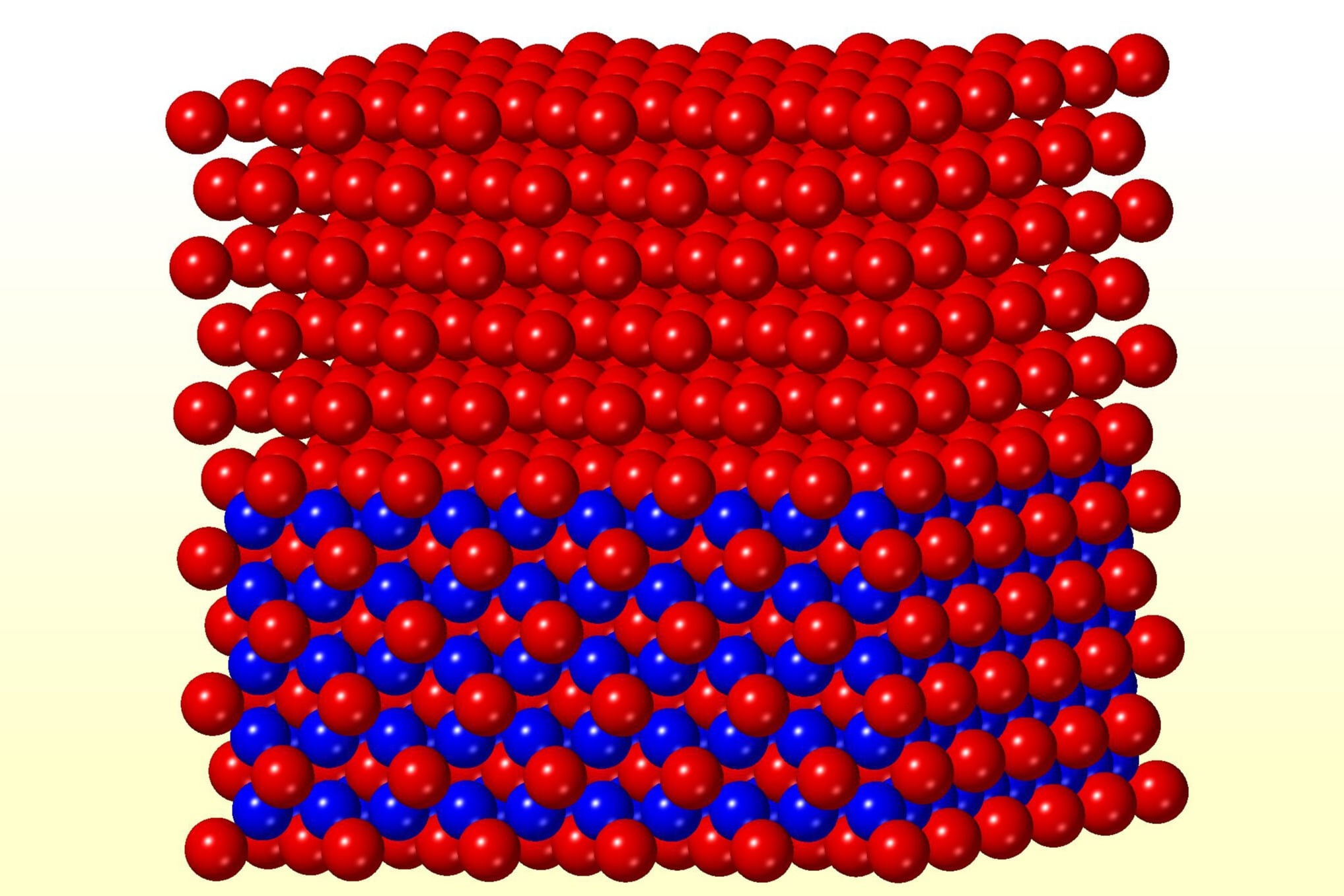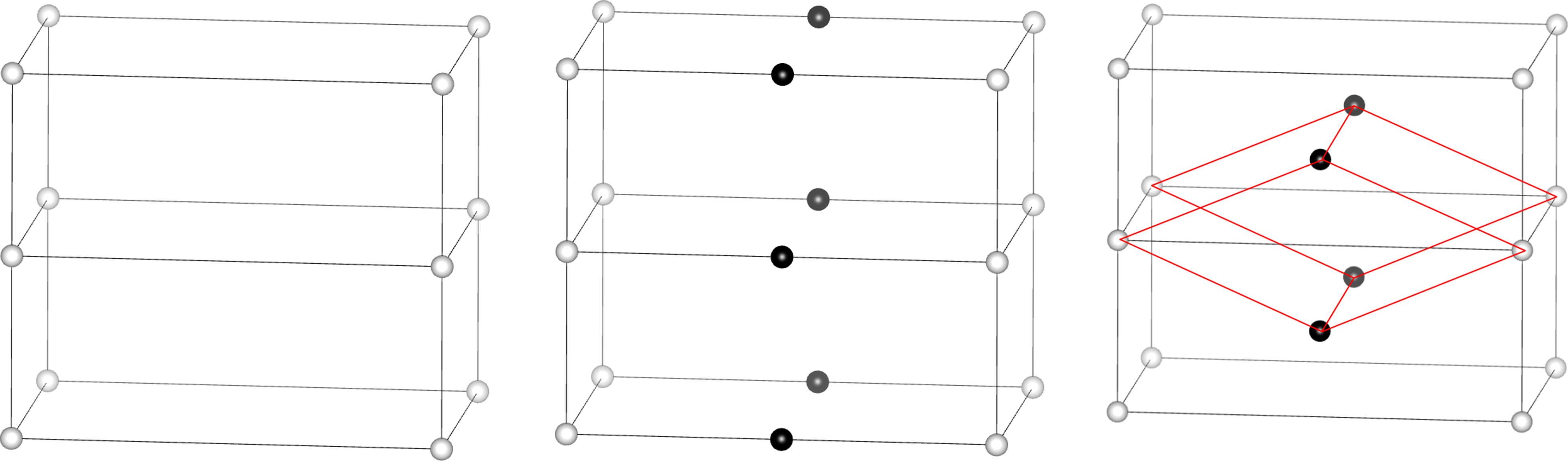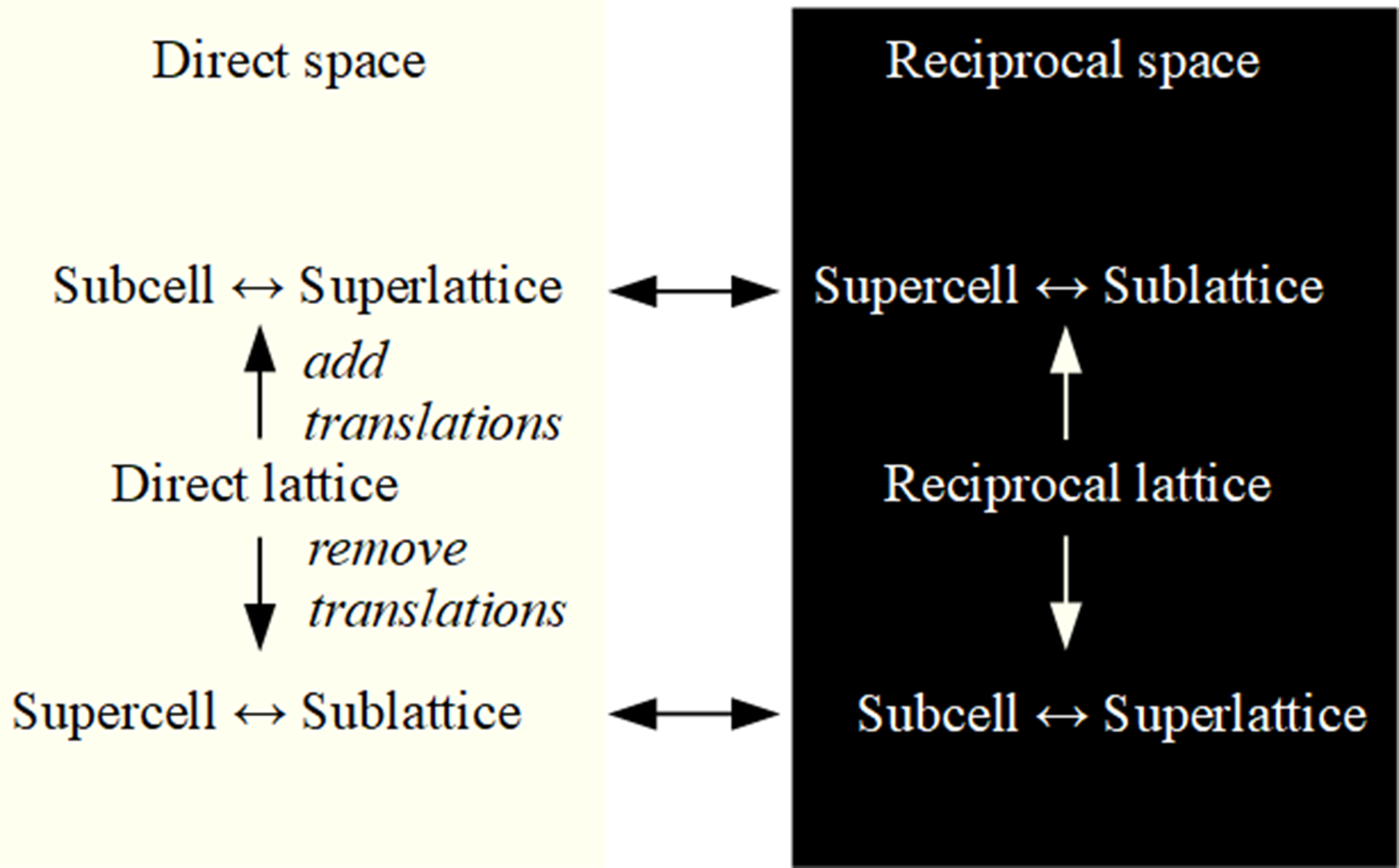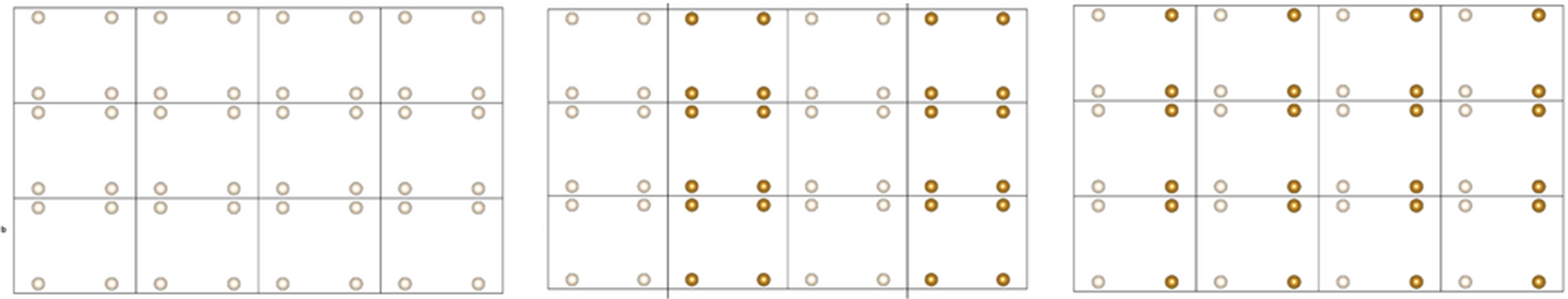•# The super–sub schizophrenia

Massimo NespoloIn the previous issue of the IUCr Newsletter, I drew the reader's attention to the difference between a crystal structure and its (Bravais) lattice. This was needed because of the surprisingly widespread misunderstanding that shows up in so many textbooks, articles and lectures. This time we dig a little deeper and try to see what happens when “something” is added to or removed from, in a systematic way, a lattice and a structure.

First of all, let us consider a lattice, i.e. the mathematical abstraction made up of zero-dimensional points (the lattice nodes) that represents the periodicity of a crystal structure (remember, there are no atoms in a lattice). To a lattice, one can only add lattice nodes; similarly, from a lattice, one can only remove lattice nodes. This may at first just seem a mind game but we are going to see its physical meaning.

What does it mean to add to a lattice (let us call it L1 for simplicity) a set of nodes so that one obtains another lattice (let us call it L2)? If you choose one lattice node as the origin (it does not matter which you choose, they are all equivalent), then you can draw an infinite set of vectors from that origin to all the other lattice nodes. Each of these vectors represents a translation operation and all these translations do form a mathematical group: the translation group of L1 (which is a subgroup of the space group of the structure whose lattice is L1). When you add lattice nodes, you add further vectors, i.e. further translation operations. These, together with the translations of L1, again form a group and, because the order of the group (number of operations) has increased, it is actually a supergroup of that of L1. It should therefore come as no surprise that L2 is a superlattice of L1.

Now, let us think about the unit cells of the two lattices, and in particular the primitive unit cells. Because of the additional nodes in L2 with respect to L1, the primitive unit cell of L2 is smaller than the primitive unit cell of L1 and is therefore a subcell (Figure 1). So, we get the surprising result that the primitive unit cell of the superlattice is a subcell of that of the original lattice.Figure 1. Primitive unit cell of the original lattice L1 (left) and of two superlattices L2 obtained by adding a second (infinite) set of lattice nodes (black) obtained from the original by translations 0,½,0 (middle) and 0,½,½ (right). Axes: a is towards the observer, b is horizontal and c is vertical. The figure shows two unit cells of L1 along the c axis. The primitive unit of the superlattice in the middle has its edges parallel to that of L1 but its translation vector along b is half of that of L1. The primitive unit cell of the superlattice on the right is outlined in red.

Let us next do the opposite: remove a subset of lattice nodes from L1 so that the remaining lattice nodes still form a lattice L2. The set of translation vectors of L2 is a subset of that of L1; therefore, the group of translations of L2 is a subgroup of the group of translations of L1. As a consequence, L2 is a sublattice of L1, whereas its primitive unit cell is a supercell of that of L1 (Figure 1 applies again, this time by considering the middle and right parts as representing L1, the left part representing L2). So now we learn that a sublattice consists of supercells.

In the literature, this simple relation is quite often misunderstood. If the primitive unit cell of L2 is larger than that of L1, i.e. if it is a supercell, then L2 is itself often, but incorrectly, called a “superlattice”; it is actually a sublattice, because it has lost symmetry operations (in particular, translations) with respect to L1. Quite obviously the relation sub–super is reversed in going from one space to its dual (direct ↔ reciprocal). A simple graphic guide to avoid this type of mistake is provided in Figure 2.Figure 2. The relation between a lattice and its super/sublattices in the dual spaces.

When one sees extra diffraction spots (“extra” with respect to what one expects to see…) in the diffraction pattern, one speaks of superlattice diffractions, which is perfectly consistent, considering that the periodicity in the reciprocal lattice has increased. However, the crystal structure that has caused these extra diffractions corresponds to a sublattice in direct space. So, when talking about diffraction (reflections, peaks) one uses the super–sub terminology for reciprocal lattices rather than for direct lattices.

Let us now move to the structure (built by atoms) and analyse the meaning of superstructure and substructure.

In a crystal structure some atomic positions may statistically be occupied by different types of atoms (let us say two, for the sake of simplicity). This often occurs in inorganic and mineral structures, where atoms are disordered at positions that are equivalent under the operations of the space group. Under some changes of the external conditions (temperature, pressure, applied field, etc.) the disorder distribution may no longer be stable and a phase transition may occur, after which different atoms become ordered. The structure with a disordered atomic distribution is called a basic structure. The positions that were equivalent before the transition are no longer equivalent afterwards. Depending on whether the ordering does or does not involve a reduction in the translational symmetry (i.e. whether or not the primitive unit cell is transformed to a supercell), the resulting structure is called a superstructure or a derivative structure (Figure 3). Note that, because the unit cell of the superstructure is a supercell of the unit cell of the basic structure, its lattice is actually a sublattice of that of the basic structure!Figure 3. A simple example of a basic structure (left), superstructure (middle) and derivative structure (right).

The term superstructure is also used in a different, although not unrelated, meaning when studying modular structures. A crystal structure is built by the periodic and regular repetition of a structural element: a molecule (in the case of molecular crystals), a set of atoms or ions forming a coordination polyhedron (in the case of a non-molecular crystal, e.g. NaCl) or an extended network (like in the case of metals). In some cases, one can ideally cut the crystal structure into bigger and more complex building blocks: layers (sheets), rods (chains) or finite modules composed of a number of atoms significantly larger than those identifying a coordination polyhedron. These crystals are known as modular structures, and can be seen as superstructures with respect to the modules. Here another common mistake must be pointed out. The modules are subperiodic, i.e. they are periodic in a subspace of three-dimensional space (diperiodic, monoperiodic or nonperiodic depending on whether the module is a layer, a rod or a finite block) but they are not “low-dimensional”. The expression “low-dimensional material” that today pollutes scientific literature is a semantic nonsense.

The last concept to consider is the substructure. A crystal structure is composed of N ≥ 1 atoms in the asymmetric unit and all those equivalent, obtained by applying the symmetry operations of the space group. Stated in an equivalent and more general way, remembering that the infinite set of atoms equivalent under the action of the space group is called a crystallographic orbit, a crystal structure is composed of N ≥ 1 crystallographic orbits. When N > 1, one can consider a subset M (1 ≤ M < N) of the crystallographic orbits, and obtain, in this way, a substructure. Now, when one considers only the periodicity of a substructure, one gets the lattice of this substructure. Its unit cell can either coincide with that of the whole structure or be a subcell. Therefore, the lattice of a substructure either coincides with the lattice of the original structure or is a superlattice of it: it can never be a sublattice. Despite the fact that these concepts and definitions sound crystal (!) clear, many publications call a substructure “sublattice”, which not only is plain wrong even when considering the lattice of the substructure, but also shows, once more, the deep-rooted confusion between structure and lattice that we analysed in the last contribution. Here is one recent example, but one can easily find a lot more by a simple keyword search on the World Wide Web. It is to be emphasized that this sloppy language is quite common in high-impact journals, such as in the example above: impact factors are by no means a guarantee of sound science. IUCr journals of course do not fall into this sort of trap!

I conclude this short survey by pointing out that is not uncommon to find serious mistakes occurring even in official definitions provided by non-specialists. For example, the entry superlattice in the IUPAC Gold Book represents a double mistake: because it actually deals with the concept of superstructure and because the lattice of this superstructure is a sublattice. It is to be noted that the same IUPAC Gold Book does not have any entry for “sublattice”, “superstructure” or “substructure”. It is highly regrettable that societies in other fields take the initiative of providing definitions without checking whether they are correct with the specialists. We can only hope that in the future they will check with the reference for crystallographic terms, which is the Online Dictionary of Crystallography.

### References

Buerger, M. J. (1947). J. Chem. Phys. 15, 1–16.

Ferraris, G., Makovicky, E. & Merlino, S. (2008). Crystallography of Modular Materials, 2nd ed., p. 384. Oxford University Press.

Nespolo, M. (2019). J. Appl. Cryst. 52, 451–456.

11 September 2019